# AP Biology : Population Genetics

## Example Questions

### Example Question #21 : Population Genetics

Which of the following are assumptions made by the Hardy-Weinberg principle?

Random mating and sexual reproduction

All of these

Large population size

No migration, mutation, and selection

All of these

Explanation:

The Hardy-Weinberg principle is a theory that describes how allele frequencies may change within a population absent of evolutionary mechanisms. The theorem is based on certain assumptions regarding the population in question. These assumptions include random mating, large population size, sexual reproduction, and the absence of migration, mutation and selection. It is exceedingly rare for all the Hardy-Weinberg assumptions to be met in nature, but this theory is a tool used to study allele frequencies within populations.

### Example Question #21 : Population Genetics

In the Hardy-Weinberg theorem,refers to what?

The frequency of the recessive allele

The frequency of the homozygous dominant genotype

The frequency of the dominant allele

The frequency of the homozygous recessive genotype

The frequency of the dominant allele

Explanation:

In the Hardy-Weinberg theory, the two equations used are: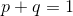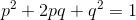Here,refers to the frequency of the dominant allele and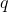refers to the frequency of the recessive allele. Subsequently,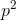refers to the frequency of the homozygous dominant genotype,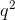refers to the frequency of the homozygous recessive genotype, and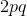refers to the frequency of the heterozygous genotype.

### Example Question #22 : Population Genetics

Which of the following are deviations from the Hardy-Weinberg equilibrium?

Mutation only

Random mating only

Migration only

Mutation and migration

Random mating and mutation

Mutation and migration

Explanation:

In Hardy-Weinberg equilibrium, deviations are violations of the assumptions of the Hardy-Weinberg theory. The assumptions of the Hardy-Weinberg theoru include random mating, large population size, sexual reproduction, and the absence of migration, mutation and selection. Therefore, deviations from the Hardy-Weinberg equilibrium from the options are mutation and migration.

### Example Question #21 : Understanding Hardy Weinberg Assumptions And Calculations

What is the best explanation for a population being described as an evolutionary unit?

Natural selection only occurs across species

Genetic changes occur only in populations

Populations can evolve, not individuals

A population's gene pool remains the same throughout time

Natural selection only involves individuals, not populations

Populations can evolve, not individuals

Explanation:

The genetic make up of populations can be measured over time. While genetic changes only occur at the individual level, it is only populations that evolve since genetic changes take many generations to develop into phenotypic changes that may be observed as changing allele frequencies over time.

### Example Question #21 : Population Genetics

Which of the following is not an assumption of Hardy-Weinberg equilibrium?

Mutations do not occur

There is no flow of new genes from an outside population

The population is small

Mating is random

No traits have an influence on natural selection

The population is small

Explanation:

The five answers listed are all assumptions of Hardy-Weinberg equilibrium except for the population being small. In fact, the population must be large so that random chance during mating and death will not result in certain alleles changing their proportion in the population.

### Example Question #21 : Understanding Hardy Weinberg Assumptions And Calculations

In a population of rabbits, the dominant allele for eye color is black and the recessive allele for eye color is brown. If 9% of the population is brown eyed, what percent of the population is heterozygous for eye color?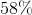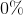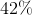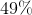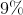Explanation:

In order to answer this question, you must remember the Hardy-Weinberg equations.In the above equations,stands for the frequency of the dominant allele andstands for the frequency of the recessive allele.

It is also important to realize thatstands for the homozygous dominant population,stands for the homozygous recessive population, andstands for the heterozygous population.

We are told that 9% of the population is brown eyed, or homozygous recessive. Let's solve for.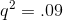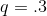Now let's solve for.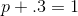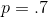The question asks for the percent of the population that is heterozygous. In order to do this, we look at the first equation and plug in known values.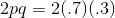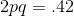### Example Question #27 : Population Genetics

Under the Hardy-Weinberg Equilibrium Principle, allelic and genotype frequencies do not change in an ideal population, but this assumption is only valid under certain conditions.

A population must meet all of the following conditions to be in Hardy-Weinberg equilibrium except __________.

Mating must be random

natural selection must occur

no migration

no mutations

no natural selection

natural selection must occur

Explanation:

Under the Hardy-Weinberg Principle, there is no change in the frequency of alleles or genotypes in a population because there are no changes to the population, such as: natural selection, mutations, migration, or chance events. In addition, mating must be random. Therefore, is it easy to see that most populations do not meet the requirements of this principle.

### Example Question #21 : Understanding Hardy Weinberg Assumptions And Calculations

Eye color in a certain species is decided by a single gene locus. Only two alleles influence eye color in a population of this species that exists in Hardy-Weinberg equilibrium. The dominant allele codes for brown eyes, while the recessive allele codes for blue eyes.

If the frequency of the brown allele is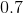, what percent of the population is heterozygous at this locus?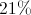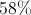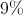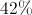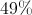Explanation:

For problems of this type, we need to understand the Hardy-Weinberg equations:Here,represents the frequency of the dominant allele, while c refers to the frequency of the recessive allele.anddenote the proportion of homozygous dominant and recessive phenotypes, respectively. Finally, the proportion of heterozygotes is denoted by.

We already know that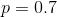, and if only two alleles are present in the population,must be equal to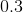.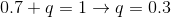Using the values forand, we can solve for the proportion of heterozygotes using theterm of the Hardy-Weinberg equation.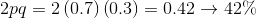### Example Question #201 : Evolution And Genetics

Gene flow occurs __________.

when natural forces act on existing variation

when new individuals are added to a population

when a population is split

when two populations mix, but do not mate

when new individuals are added to a population

Explanation:

Gene flow occurs when new individuals of the same species are added into a population. Think of it as new genes flowing into the existing gene pool.

This influx of new genes has the potential to disrupt the allele frequency in the given population; thus, no migration (gene flow) can occur in a population in Hardy-Weinberg equilibrium.

### Example Question #1 : Understanding Genetic Drift, Bottleneck Effect, And Founder Effect

Populations do not necessarily consist of the individuals best adapted to survive and reproduce. Theoretically, the most fit individuals in a population will survive longest and reproduce the most, however chance plays a role in who survives, reproduces, and whose offspring survive to reproduce as well.

In a population where the allele frequency shifts by random chance, the mechanism of evolution at work is __________.

migration

natural selection

genetic drift

mutation